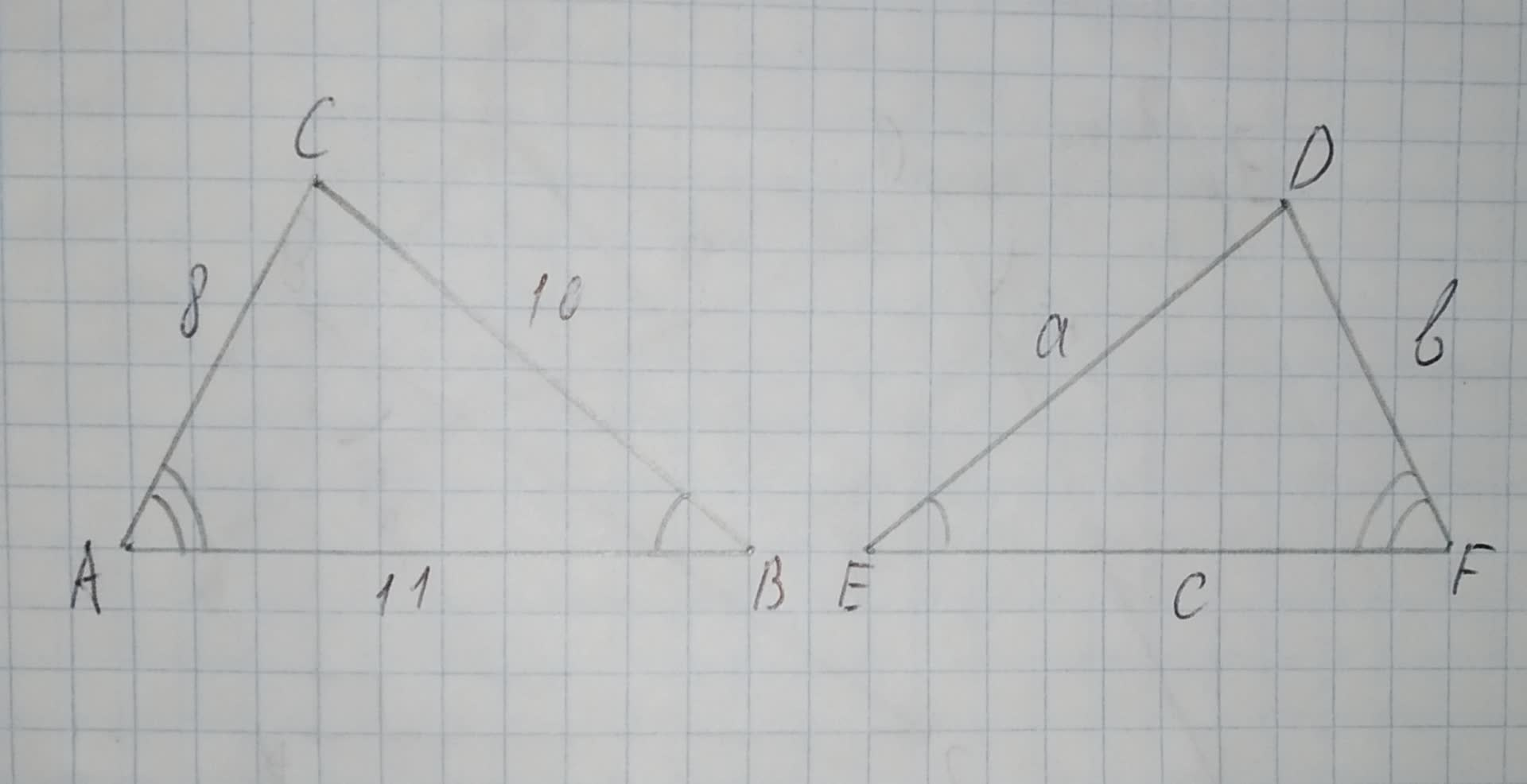# For the triangles shown, we can express their congruence with the statement /_ABC ~= /_FED. By reordering the vertices, express this congruence with a different statement. 12210203302.jpgiohanetc 2020-12-09 Answered
For the triangles shown, we can express their congruence with the statement $\mathrm{△}ABC\stackrel{\sim }{=}\mathrm{△}FED$. By reordering the vertices, express this congruence with a different statement.You can still ask an expert for help

## Want to know more about Congruence?

• Questions are typically answered in as fast as 30 minutes

Solve your problem for the price of one coffee

• Math expert for every subject
• Pay only if we can solve it2abehn
Step 1
Given- The triangles ABC and FED are congruence with $\mathrm{△}ABC\stackrel{\sim }{=}\mathrm{△}FED$ from the given figure,To express- The congruence with a different statement by reordering the vertices.
Concept Used- Two triangles are said to be congruent if the three sides and the three angles of both the angles are equal in any orientation.
Step 2
Explanation- As we know that two triangles are said to be congruent if the three sides and the three angles of both the angles are equal in any orientation.
Now, from the figure, as we see that the corresponding sides and corresponding angles are equal, so using the congruence law , we can write in correct order as,
$\mathrm{△}ABC\stackrel{\sim }{=}FED$.
So, the correct order of congruence can be written as $\mathrm{△}ABC\stackrel{\sim }{=}FED$.
Answer- Hence, the congruence with a different statement by reordering the vertices can be writtena as $\mathrm{△}ABC\stackrel{\sim }{=}FED$.